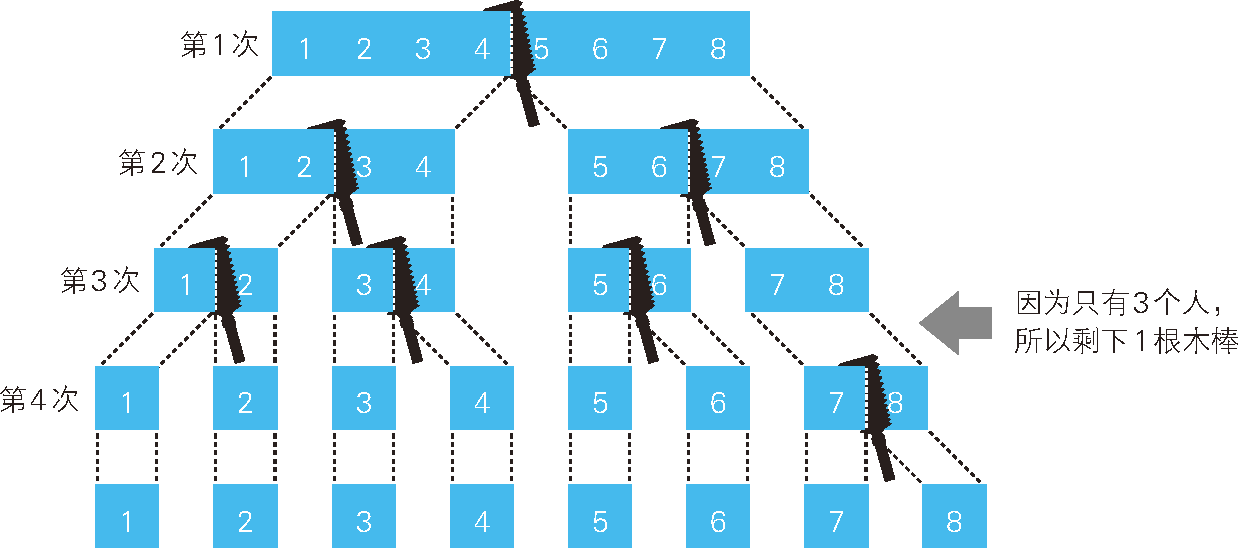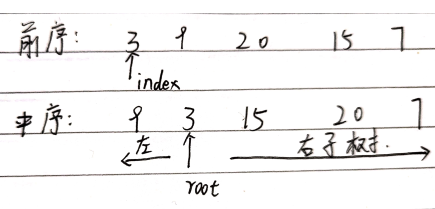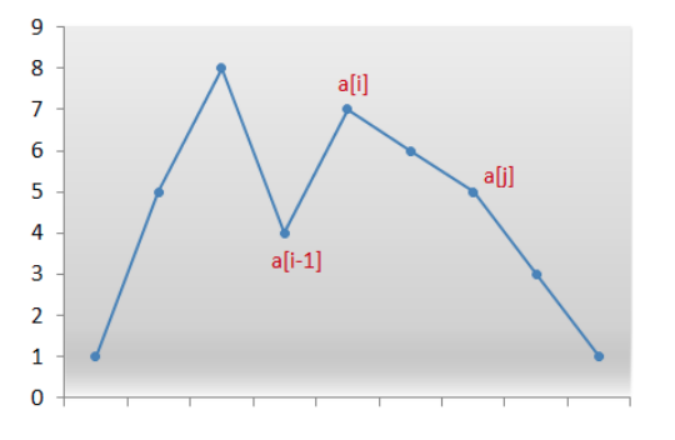• 我们发现这个题里每一种...我们从 000000000 到 333333333 来枚举的话，第一个符合条件结果当然就是所有答案中字典序最小一个了。 枚举这个排列我们需要用9个for，当然也可以用递归来实现，不过我还是喜欢...
我们发现这个题里每一种“移动套餐”用的次数只有0，1，2，3 是有效的，4和0是一样的。
所以我们开一个数组rot来记录这9个套餐分别用了多少次。
字典序的处理和我们的枚举顺序息息相关。
我们从 000000000 到 333333333 来枚举的话，第一个符合条件的结果当然就是所有答案中字典序最小的一个了。
枚举这个排列我们需要用9个for，当然也可以用递归来实现，不过我还是喜欢视觉感比较强烈的9个for。。。。
在每一种排列下，我们需要通过这些套餐的方案来计算临时结果path。
然后判断是不是所有的钟表都达到了12点。
如果是的话，我们就可以输出了。
输出的时候，要记得把套餐使用次数利用上。。。

#include <iostream>
using namespace std;

int clocks;//记录输入的
int rot; //记录转动次数

int main(int argc, char const *argv[])
{

for (int i = 1; i <= 9; ++i){
cin>>clocks[i];
}
int path={0};

for (rot = 0; rot < 4; ++rot)
for (rot = 0; rot < 4; ++rot)
for (rot = 0; rot < 4; ++rot)
for (rot = 0; rot < 4; ++rot)
for (rot = 0; rot < 4; ++rot)
for (rot = 0; rot < 4; ++rot)
for (rot = 0; rot < 4; ++rot)
for (rot = 0; rot < 4; ++rot)
for (rot = 0; rot < 4; ++rot){
//枚举出一种全体转动情况 一共有4^9种
//根据转动情况 确定结果
path = (clocks + 3*(rot + rot + rot )) % 12;
path = (clocks + 3*(rot + rot + rot + rot )) % 12;
path = (clocks + 3*(rot + rot + rot )) % 12;
path = (clocks + 3*(rot + rot + rot + rot )) % 12;
path = (clocks + 3*(rot + rot + rot + rot + rot )) % 12;
path = (clocks + 3*(rot + rot + rot + rot )) % 12;
path = (clocks + 3*(rot + rot + rot )) % 12;
path = (clocks + 3*(rot + rot + rot + rot)) % 12;
path = (clocks + 3*(rot + rot + rot )) % 12;

int sum = 0;
for (int i = 1; i <= 9 ; ++i)
sum += path[i];//若全是12 则全是0
if(!sum){
//找到了第一个解 也就是字典序的最小解
//输出解
for (int i = 1; i <= 9; ++i)
{
for (int j = 0; j < rot[i]; ++j)
{
//rot存的是个数
cout<<i<<" ";
}
}
cout<<endl;
return 0;
}

}

return 0;
}

转载于:https://www.cnblogs.com/yuchenlin/p/sjtu_oj_1047.html
展开全文• ## 回溯法与枚举法

千次阅读 2018-08-30 10:42:53
回溯解决问题有全排列，全组合，枚举什么。 比如，给定一串不重复字母，让你按字典顺序进行排列，这是个全排列问题，很好理解，但编程很麻烦。如果给定字母可以重复，让你按字典的顺序排列，这个问题...
1 回溯法应用

回溯法，顾名思义，生活中有一类人，很执拗，比如我，不到黄河心不死，到了黄河怎么办？往回走呗，难不成跳下去？

回溯法解决的问题有全排列，全组合，枚举什么的。

比如，给定一串不重复字母，让你按字典顺序进行排列，这是个全排列的问题，很好理解，但编程很麻烦。如果给定的字母可以重复，让你按字典的顺序排列，这个问题是不是更复杂了呢？

回溯法，跟数一样，随机取出一个元素，此时情况有N种，则有N个这有的节点，然后在剩下的集合中，又随机取个数，此时情况可能有N-1种（可能小于N-1如果有重复的项），重复上面的生成节点的过程。当处理到最后一个元素或者当前的路径以及不满足要求了，就往回走了，递归回来。

回溯构建数的时候，取出了那个元素，下一个集合就没有这个元素了。

2 回溯法固化模板

回溯法就相当于搜索一棵树，使用深度优先搜索数的方法。

def dfs(输入):
if 当前状态为边界：
记录或输出
return

for i in range(len(输入))：#横向遍历所有子节点
目前遍历的当前子节点
if 子状态满足约束条件：
dfs(子状态)



3 题集

3.1 字符串排列（全排列问题）

题目描述

输入一个字符串,按字典序打印出该字符串中字符的所有排列。例如输入字符串abc,则打印出由字符a,b,c所能排列出来的所有字符串abc,acb,bac,bca,cab和cba。

解题思路：字典排序，重复元素的全排列。深度优先搜索，即为空是返回．先从Ｎ个样本中取一个，接在递归在剩下的样本中取，为什么要递归？因为这样再取完以后会退回到上一级．

重复原始处理，排好序后，如果取到的这个，和上个相似，就continue．

假设根就是空，第一层会有N个子节点，对重复的取一个X，然后从集合中去掉取出的这一个X（重复的也去掉一个），迭代。

class Solution:
def Permutation(self, ss):
if not ss:
return []
ss = sorted(ss)
temp = []
N = len(ss)
def dfs(ss,cur):
if not ss:
if len(cur) == N:
temp.append(cur)
return
for i in range(len(ss)):
if i > 0:
if ss[i] == ss[i-1]:
continue
if len(ss) == N:
cur = ss[i]
else:
cur = cur+ss[i]
if i+1 < len(ss) and i>0:
ss1 = ss[:i]+ss[i+1:]
elif i==0:
ss1 = ss[i+1:]
else:
ss1 = ss[:i]
dfs(ss1, cur)
dfs(ss,'')
return temp

3.2 矩阵中的路径

题目描述

请设计一个函数，用来判断在一个矩阵中是否存在一条包含某字符串所有字符的路径。路径可以从矩阵中的任意一个格子开始，每一步可以在矩阵中向左，向右，向上，向下移动一个格子。如果一条路径经过了矩阵中的某一个格子，则之后不能再次进入这个格子。 例如 a b c e s f c s a d e e 这样的3 X 4 矩阵中包含一条字符串"bcced"的路径，但是矩阵中不包含"abcb"路径，因为字符串的第一个字符b占据了矩阵中的第一行第二个格子之后，路径不能再次进入该格子。

解题思路：首先输入的是字符串‘ csadee’，需要映射成矩阵（嗯，绕了很长时间）。因为起始位置是随机的，所以需要遍历所有的矩阵作为起始位置。为了节约时间，我们需要剪枝，即如果当前位置的值不等于path，那就可以直接返回。

还需要注意一个约束，就是不能往回走，最开始我的代码是：

class Solution:
def __init__(self):
self.flag = False
def hasPath(self, matrix, rows, cols, path):
if not matrix:
return False
for j in range(rows):
for i in range(cols):
self.hasp(matrix,j,i,path[:], rows)
if self.flag:
return True
return False

def hasp(self,matrix, curr, curc, path, rows, cols):
if not path:
self.flag = True
return
if curr<0 or curc<0 or curr>rows-1 or curc>cols-1:
return
index = curr * cols + curc
if matrix[index] == path:

self.hasp(matrix, curr-1, curc, path[1:], rows, cols)

self.hasp(matrix, curr + 1, curc, path[1:], rows, cols)

self.hasp(matrix, curr , curc - 1, path[1:], rows, cols)

self.hasp(matrix, curr , curc + 1, path[1:], rows, cols)

本地跑属于False的也会返回True，什么原因呢？因为我重复了曾经的路径，贴上正确的代码：

class Solution:
def __init__(self):
self.flag = False
def hasPath(self, matrix, rows, cols, path):
if not matrix:
return False
for j in range(rows):
for i in range(cols):
self.hasp(matrix,j,i,path[:], rows, cols,[(j,i)])
if self.flag:
return True
return False

def hasp(self,matrix, curr, curc, path, rows, cols,history):
if not path:
self.flag = True
return
if curr<0 or curc<0 or curr>rows-1 or curc>cols-1:
return
index = curr * cols + curc
if matrix[index] == path:
if (curr-1, curc) not in history:
self.hasp(matrix, curr-1, curc, path[1:], rows, cols,history+[(curr-1, curc)])
if (curr + 1, curc) not in history:
self.hasp(matrix, curr + 1, curc, path[1:], rows, cols,history+[(curr + 1, curc)])
if (curr , curc-1) not in history:
self.hasp(matrix, curr , curc - 1, path[1:], rows, cols,history+[(curr, curc-1)])
if (curr , curc+1) not in history:
self.hasp(matrix, curr , curc + 1, path[1:], rows, cols,history+[(curr , curc+1)])

3.2 矩阵中的路径

题目描述

地上有一个m行和n列的方格。一个机器人从坐标0,0的格子开始移动，每一次只能向左，右，上，下四个方向移动一格，但是不能进入行坐标和列坐标的数位之和大于k的格子。 例如，当k为18时，机器人能够进入方格（35,37），因为3+5+3+7 = 18。但是，它不能进入方格（35,38），因为3+5+3+8 = 19。请问该机器人能够达到多少个格子？

解题思路：数位之和，我想到的就是把它转换成str，然后按位读取str，转换成int并求和。感觉这道题很简单啊，思路也对，时间复杂度总是超了。我原来的思路跟上道题一样，边界条件改了（超出矩阵和数位之和），其实条件就只为（0,0）。调了很久，后来知道是走过的路径应该设置为全局变量。当初没有设置为全局变量。

正确代码：

class Solution:
def movingCount(self, threshold, rows, cols):
self.row, self.col = rows, cols
self.dict = []

self.search(threshold, 0, 0)
return len(self.dict)

def search(self, threshold, i, j):
ss = 0
for k in str(i)+str(j):
ss = ss+int(k)
if ss > threshold:
return
self.dict.append((i,j))
if i != self.row - 1 and (i+1,j) not in self.dict:
self.search(threshold, i + 1, j)
if j != self.col - 1 and (i,j+1) not in self.dict:
self.search(threshold, i, j + 1)


展开全文• 个整数随机选取任意多个，每种方案里数从小到大排列，按字典序输出所有可能选择方案。 输入: 4 输出: 1 1 2 1 2 3 1 2 3 4 1 2 4 1 3 1 3 4 1 4 2 2 3 2 3 4 2 4 3 3 4 4 方法流程: 设置预存数组 设置递归...
 指数型枚举组合型枚举排列型枚举字符串排列规定人数木条切割前序中序遍历重建二叉树打印从1到最大的n位数(全排列问题)
指数型枚举

从 1−𝑛 这 𝑛 个整数中随机选取任意多个，每种方案里的数从小到大排列，按字典序输出所有可能的选择方案。
输入:
4
输出:
1
1 2
1 2 3
1 2 3 4
1 2 4
1 3
1 3 4
1 4
2
2 3
2 3 4
2 4
3
3 4
4

方法流程:

设置预存数组
设置递归函数:(从第几个数开始, 从数组第几位存起)

法一: 单参数递归体现回溯感
#include <iostream>
using namespace std;
int n, num, id;

void p() {
for (int i = 0; i <= id; i++) {
i == 0 || cout << " ";
cout << num[i];
}
cout << endl;
return ;
}

void func(int s) {
// 从s起始的升序循环枚举
for (int i = s; i <=n; i++) {
num[id] = i;
p();
cnt++;
// 从下一个数开始,从下一位存起
func(s + 1);
// 回溯, 为下个数,从这位存起做准备
cnt--;
}
}

int main() {
cin >> n;
// 从第一个数开始
func(1);
return 0;
}

法二: 双参数递归减少全局变量
#include <iostream>
using namespace std;
int n, num;

void p(int id) {
for (int i = 0; i <= id; i++) {
i == 0 || cout << " ";
cout << num[i];
}
cout << endl;
return ;
}

void func(int s, int id) {
// 从s起始的升序循环枚举
for (int i = s; i <= n; i++) {
// 起始数保存
num[id] = i;
// 打印枚举序列
p(id);
// 下一存存储位的递归
func(i + 1, id + 1);
}
return ;
}

int main() {
cin >> n;
// 从第一个数开始, 从数组第一位存起
func(1, 0);
return 0;
}

组合型枚举

从 1−𝑛 这 𝑛 个整数中随机选取 𝑚 个，每种方案里的数从小到大排列，按字典序输出所有可能的选择方案。
输入:
5 3
输出:
1 2 3
1 2 4
1 2 5
1 3 4
1 3 5
1 4 5
2 3 4
2 3 5
2 4 5
3 4 5

方法流程:
仅比指数型枚举多一个判断条件: 凑够m个数再输出
需要额外设置一个left待选余量

从第几个数开始选
存入第几个位置
还要选几个

法一: 双参数递归体现回溯感
#include <iostream>
using namespace std;
int n, m, num, id;

void p() {
for (int i = 0; i < id; i++) {
i == 0 || cout << " ";
cout << num[i];
}
cout << endl;
return ;
}

// 从第s个数开始选, 还需选left个数
void func(int s, int left) {
// 选够m个数再打印
if (left == 0) {
p();
return ;
}
// 从s起始的循环枚举
for (int i = s; i <= n; i++) {
num[id] = i;
id++;
func(i + 1, left -1);
id--;
}
return ;
}

int main() {
cin >> n >> m;
// 从第1个数开始选, 还需选m个数
func(1, m);
return 0;
}

法二: 三参数递归减少全局变量(略)
排列型枚举

从 1−𝑛 这 𝑛 个整数排成一排并打乱次序，按字典序输出所有可能的选择方案。
输入:
3
输出:
1 2 3
1 3 2
2 1 3
2 3 1
3 1 2
3 2 1

方法流程:
每次循环都为n个数, 每选够n个数再输出
为了避免重复,需要添加标记数组
法一: 单参数递归更新存储索引
#include <iostream>
using namespace std;
// 防止排列时重复选取, 设计标记数组
int n, num, mark;

void p() {
for (int i = 0; i < n; i++) {
i == 0 || cout << " ";
cout << num[i];
}
cout << endl;
return ;
}

void func(int id) {
// 存够n个输出一波
if (id == n) {
p();
return ;
}
// 让各个元素在相同位置进行一次记录
for (int i = 1; i <= n; i++) {
// 若元素已被记录则不再重复记录
if (mark[i] == 0) {
// 已被记录的标记
mark[i] = 1;
num[id] = i;
// 记录下一位
func(id + 1);
// 回溯去标记,以便其他递归线中可以再存
mark[i] = 0;
}
}
return ;
}

int main() {
cin >> n;
// 从第零位开始记录
func(0);
reutrn 0;
}

法二: 单参数递归记录余量, 动态调整存储索引体现回溯感(略)
字符串排列
规定人数木条切割
来源: Leetcode2020跨年 : Q04 切分木棒.

假设要把长度为 n 厘米的木棒切分为 1 厘米长的小段，但是 1 根木棒只能由 1 人切分，当木棒被切分为 3 段后，可以同时由3个人分别切分木棒。 求最多有 m 个人时，最少要切分几次。譬如 n ＝ 8，m＝ 3 时如图所示，切分 4 次就可以了。#include <iostream>
int n, m, curr;

// m个人要切n段木头, 目前木头有curr段
int cutbar(int n, int m, int curr) {
// 已达成目标, 本次过程为0,不算
if (curr >= n) return 0;
// 人多木段少, 木段数翻倍, 过程 + 1
if (curr < m) return 1 + cutbar(n, m, curr * 2);
// 木段数增加人的数量, 过程 + 1
else return 1 + cutbar(n, m, curr + m);
}

int main() {
cin >> m >> n;
cout << cutbar(n, m, 1) << endl;
}

前序中序遍历重建二叉树

输入某二叉树的前序遍历和中序遍历的结果，请重建该二叉树。假设输入的前序遍历和中序遍历的结果中都不含重复的数字。
输入: (两个数组)
前序遍历 preorder = [3,9,20,15,7]
中序遍历 inorder = [9,3,15,20,7]
输出:(一个TreeNode指针)
… .3
… ./ \
…9  20
… … /  \
… .  15   7

题解参考链接.思路:

以前序数组开头为基准, 在中序数组中寻找每一个父结点
更新前序数组开头, 先后对中序数组左右侧进行递归操作

/*
struct TreeNode {
int val;
TreeNode *left;
TreeNode *right;
// 构造函数
TreeNode(int x) : val(x), left(NULL), right(NULL) {}
};
*/

class Solution {
public:
// 用于记录前序遍历的索引
int index = 0;

// left, right 为 中序数组子树片段的左右边界
TreeNode* recursionBuild(vector<int>& preorder, vector<int>& inorder, int left, int right) {
// 两个递归终止条件
// 1. 前序数组遍历结束
// 2. 中序数组子树片段为空
if (index == preorder.size() || left == right) {
return NULL;
}
TreeNode* Head = NULL;
// 在中序子树片段中寻找对应的前序根结点
for (int i = left; i < right; i++) {
if (preorder[index] == inorder[i]) {
// 更新前序
index++;
// 子树根结点赋值
Head = new TreeNode(inorder[i]);
// 左子树递归
Head->left = recursionBuild(preorder, inorder, left, i);
// 右子树递归
Head->right = recursionBuild(preorder, inorder, i + 1, right);
// 已获得该树,跳出循环
break;
}
}
return Head;
}

TreeNode* buildTree(vector<int>& preorder, vector<int> inorder) {
int left = 0;
int right = preorder.size();
return recursionBuild(preorder, inorder, left, right);
}
}

打印从1到最大的n位数(全排列问题)
#include <iostream>
#include <string>
using namespace std;

vector <int> output;

// 保存排列结果
void inputNumbers(string s) {
bool isZero = true;
string temp = "";
for (int i = 0; i < s.length(); i++) {
if (isZero = true && s[i] != '0') isZero = false;
if (!isZero) temp += s[i];
}
if (temp != "") output.push_back(stoi(temp));
}

// 第pos位的递归排列
void permutation(string& s, int length, int pos) {
// 递归终止: 存够了足够的位数
if (pos == length) {
// 字符数组转为整数
intputNumbers(s);
return ;
}
for (int i = 0; i <= 9; i++) {
s[pos] = i + '0';
permutaion(s, length, pos + 1);
}
return ;
}

// 字符串的初始化与最高位的循环赋值
vector<int> printNumbers(int n) {
if (n <= 0) return vector<int>(0);
// 初始化字符数
string s(n, '0');
for (int i = 0; i <= 9; i++) {
s = i + '0';
// 从0开始的排列递归
permutation(s, s.length(), 1);
}
return output;
}

int main() {
int n;
cin >> n;
vector<int> ans = printNumbers(n);
return 0;
}



展开全文• 输入n个数，按照字典序从小到大顺序输出前n个数所有排列。 核心代码： void print_permutation(int n, int*a,int cur) { int i,j; if(cur==n) //当存入数组a数量到达n时，这时候就可以输出 { for(i =...
• 实现获取 下一个排列 函数，算法需要将给定数字序列重新排列字典下一个更大的排列。 如果不存在下一个更大的排列，则将数字重新排列成最小的排列（即升序排列）。 必须 原地 修改，只允许使用额外常数空间 ...
题目介绍

实现获取 下一个排列 的函数，算法需要将给定数字序列重新排列成字典序中下一个更大的排列。
如果不存在下一个更大的排列，则将数字重新排列成最小的排列（即升序排列）。
必须 原地 修改，只允许使用额外常数空间

力扣31题目链接：https://leetcode-cn.com/problems/next-permutation/
示例 1：

输入：nums = [1,2,3]
输出：[1,3,2]

示例2：

输入：nums = [3,2,1]
输出：[1,2,3]

示例3：

输入：nums = [1,1,5]
输出：[1,5,1]

方法一：暴力法

最简单的想法就是暴力枚举，我们找出由给定数组的元素形成的列表的每个可能的排列，并找出比给定的排列更大的排列。
但是这个方法要求我们找出所有可能的排列，这需要很长时间，实施起来也很复杂。因此，这种算法不能满足要求。
我们跳过它的实现，直接采用正确的方法。

复杂度分析

时间复杂度：O(n!)，可能的排列总计有 n! 个。
空间复杂度：O(n)，因为数组将用于存储排列。

方法二：一遍扫描
首先，我们观察到对于任何给定序列的降序排列，就不会有下一个更大的排列。例如，以下数组不可能有下一个排列：

[9, 5, 4, 3, 1]

这时应该直接返回升序排列。
所以对于一般的情况，如果有一个“升序子序列”，那么就一定可以找到它的下一个排列。具体来说，需要从右边找到第一对两个连续的数字 a[i] 和 a[i-1]，它们满足 a[i]>a[i-1]。
所以一个思路是，找到最后一个的“正序”排列的子序列，把它改成下一个排列就行了。不过具体操作会发现，如果正序子序列后没数了，那么子序列的“下一个”一定就是整个序列的“下一个”，这样做没问题；但如果后面还有逆序排列的数，这样就不对了。比如：

[1,3,8,7,6,2]
最后的正序子序列是[1,3,8]，但显然不能直接换成[1,8,3]就完事了；而是应该考虑把3换成后面比3大、但比8小的数，而且要选最小的那个（6）。接下来，还要让6之后的所有数，做一个升序排列，得到结果：
[1,6,2,3,7,8]

代码演示如下：
// 思路：从后向前找到升序子序列，然后确定调整后子序列的最高位，剩余部分升序排列
public void nextPermutation1(int[] nums){
int n = nums.length;

// 1. 从后向前找到升序子序列，找到第一次下降的数，位置记为k
int k = n - 2;
while ( k >= 0 && nums[k] >= nums[k+1] )
k --;

// 找到k，就是需要调整部分的最高位

// 2. 如果k = -1，说明所有数降序排列，改成升序排列
if ( k == -1 ){
Arrays.sort(nums);
return;
}

// 3. 一般情况，k >= 0
// 3.1 依次遍历剩余降序排列的部分，找到要替换最高位的那个数
int i = k + 2;
while ( i < n && nums[i] > nums[k] )
i ++;

// 当前的i，就是后面部分第一个比nums[k]小的数，i-1就是要替换的那个数

// 3.2 交换i-1和k位置上的数
int temp = nums[k];
nums[k] = nums[i-1];
nums[i-1] = temp;

// 3.3 k之后的剩余部分变成升序排列，直接前后调换
int start = k + 1;
int end = n - 1;
while ( start < end ){
int tmp = nums[start];
nums[start] = nums[end];
nums[end] = tmp;
start ++;
end --;
}
}

复杂度分析

时间复杂度：O(N)，其中 NN 为给定序列的长度。我们至多只需要扫描两次序列，以及进行一次反转操作。
空间复杂度：O(1)，只需要常数的空间存放若干变量。


展开全文java 算法
• 题面 题意:如果一个字符串最小表示是他自己,他就是一个Lyndon Word. ... 现在给你n,m表示用前m个字符(从a开始),拼成长度不超过n字符串,输出字典排列的的 l->r 串 l<=r<=1e7 ...
• 在之前见过回溯法中，基本上都是在枚举数字的排列，直到没有解为止，没有要求其中某一个解情况 此题就是要在这一系列解中，求出第n个解 这就涉及到了如何返回问题 先来看代码： //这道题我思路是...
• 算法思路分析：这个我能想到就只有枚举法排列组合结合了（如果里面还有更好方法一定要对话框我！！！）1、输入序列个数n，依次输入n个由abcdefghijkl乱序组成序列；2、从第j个字符开始依次与后面（j,11）...
• 任何n个字符集的排列都可以与1～nn个数字的排列一一对应，因此在此就以n个数字的排列为例说明排列的生成。n个字符全体排列之间存在一个确定线性顺序关系。所有的排列中除最后一个排列外，都有一个后继；除第...算法 integer function 扩展
• 我们中学都学过排列组合...字典是全排列生成算法最简单算法。例如：字符集{1,2,3}，如果先排较小数字，这样按字典序生成全排列有6种是：123,132,213,231,312,321。排列的公式Pn(i)=n*(n-1)......*(n-i+1)算法
•  最简单搜索莫过于枚举法，枚举整数，子串，但是在枚举过程，如果能认真分析问题本质，则可以优化问题。  晚上看了枚举排列算法，发现搜索真是太好用了。  1.生成1~n的排列  题目：输入整数n，按字典序...
• 本书旨在探讨如何优化算法效率，详细阐述了经典算法和特殊算法实现、应用技巧和复杂度验证过程，内容由浅入深，能帮助读者快速掌握复杂度适当、...15 4 排列枚举 186 15 5 正确计算 188 调试工具 191 参考文献 192
• 首先引用下《算法竞赛入门经典（第二...枚举1-10所有排列（按字典序从小到大顺序） 肯定是递归了，但是要怎么做呢？ 是不是聪明你已经想到了，就是每次把一个数放到标记数组，如果有相同数字就返回，没...
• 可参看百度文库 周源（最小表示）...题目大意：找出字典最小的排列 若通过枚举，绝对超时，参看最小表示模板 View Code #include<cstdio> ...
• 枚举全部子串（是一个排列问题，共种），判断子串是否回文。时间复杂度，空间复杂度。 （2）动态规划，同我心路。 Python源码： 我心路： 到目前为止数组、字符串题目已经做了大约十道，过程发现...
• 本科参加ACM竞赛过程积累下来一部分算法模板，和自己在PKU上面做一部分题目。 模板目录结构： 目录: 动态规划 O(n^2)最长上升子序列 nlogn最长上升子序列 高精度 计算几何 Graham扫描 两线段交点 凸...
• 1.4.3字典的两种实现方式：哈希表.二叉搜索树 1.4.4两个特殊树结构：线段树和Trie 1.5动态规划 1.5.1动态规划两种动机 1.5.2常见模型分析 1.5.3若干经典问题和常见优化方法 1.6状态空间搜索 1.6.1状态空间 1.6.2...
• 中的代码规范、简洁、易懂，不仅能帮助读者理解算法原理，还能教会读者很多实用的编程技巧。另外，书中包含的各种开发、测试和调试技巧也是在传统的语言、算法类书籍中难以见到的。 《算法竞赛入门经典》可作为...
• 中的代码规范、简洁、易懂，不仅能帮助读者理解算法原理，还能教会读者很多实用的编程技巧；书中包含的各种开发、测试和调试技巧也是传统的语言、算法类书籍中难以见到的。 《算法竞赛入门经典（第2版）》可作为...
• 1.8 检查字符串中是否包含某字符集合中的字符 15 1.9 简化字符串的translate方法的使用 18 1.10 过滤字符串中不属于指定集合的字符 20 1.11 检查一个字符串是文本还是二进制 23 1.12 控制大小写 25 1.13 访问子...
• 5.10.4 三目运算符在字符型变量中的使用 52 5.11 复杂嵌套的if语句 52 第6章 面向对象 54 6.1 面向对象程序语言的主要特征 54 6.2 类、对象和成员 55 6.3 类、对象和成员的使用方法及区别 56 6.3.1 声明一个类...
• 如何获取MSSQLServer,Oracel,Access中的数据字典信息 C#中利用GetOleDbSchemaTable获取数据库内表信息[原创] 如何解决ACCESS中SELECT TOP语句竟然返回多条记录的问题？ Asp.net 利用OleDb的GetOLEDBSchemaTable方法...
• 把指定文件中的指定位置的数字相加.cmd 把首行和尾行互换.cmd 抛弃路径尾部指定层次的字符串.cmd 拥有至高无上的特权 使用system账户.cmd 拼接相临的奇偶行文本内容.cmd 按创建时间显示完整路径.cmd 按扩展名...
• 这里有一张互联网公司面试中经常考察的问题类型总结的思维导图，我们可以结合图片中的信息分析一下。 （图片来自 leetcode) 其中算法，主要是以下几种： 基础技巧：分治、二分、贪心 排序算法：快速排序、归并...algorithm cpp interview